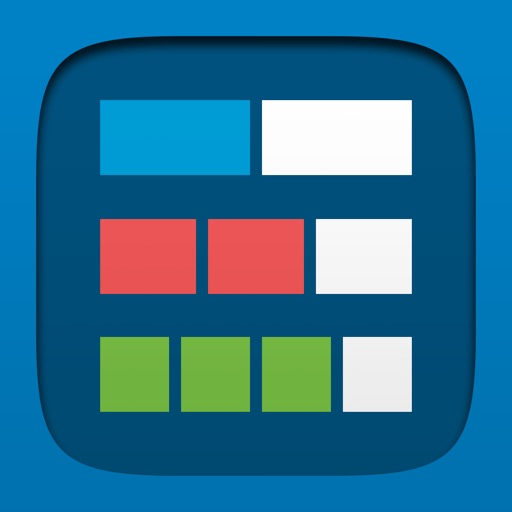## Fractions lets students use a bar or circle to represent, compare, and perform operations with fractions with denominators from 1 to 100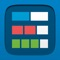# Fractions, by MLC

by The Math Learning Center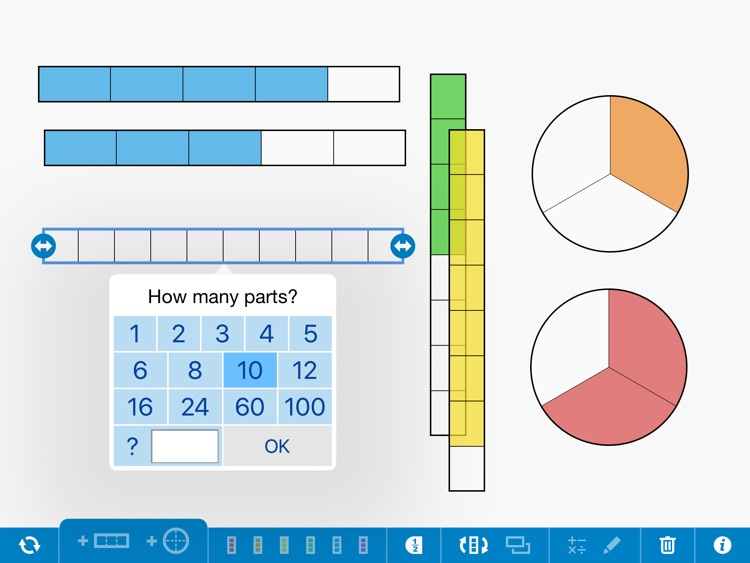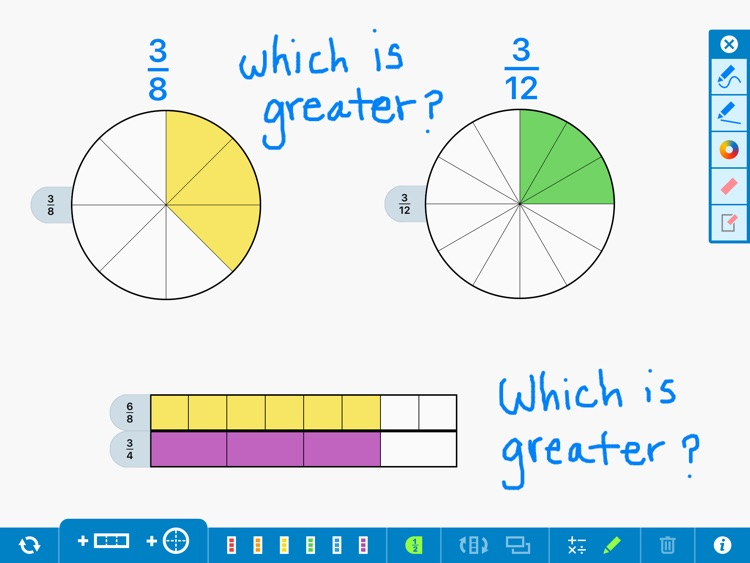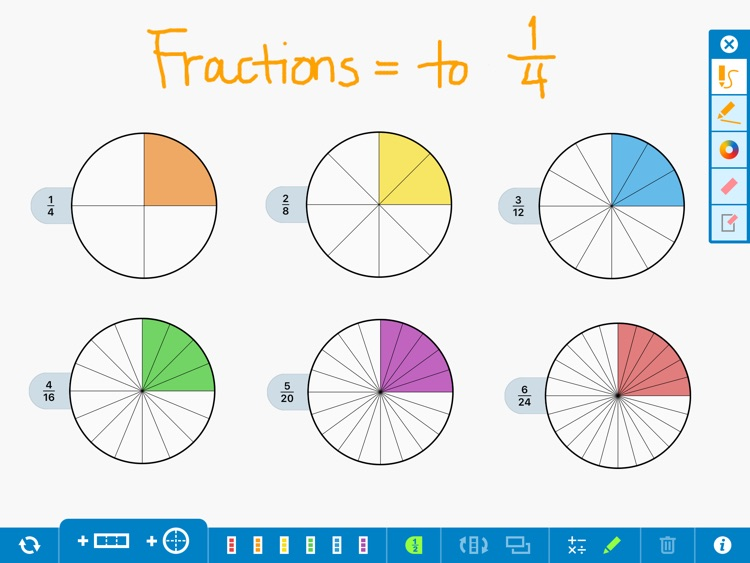Fractions lets students use a bar or circle to represent, compare, and perform operations with fractions with denominators from 1 to 100. Choose the fraction model and number of equal parts. Use a color to select specific parts to show a fraction of the whole. Reveal or hide numeric labels as needed. Superimpose fractions upon each other to compare fractions or see equal parts.Version
2.0.0
Rating
(18)
Size
2Mb
Genre
Education
Last updated
April 15, 2021
Release date
July 4, 2016

### App Screenshots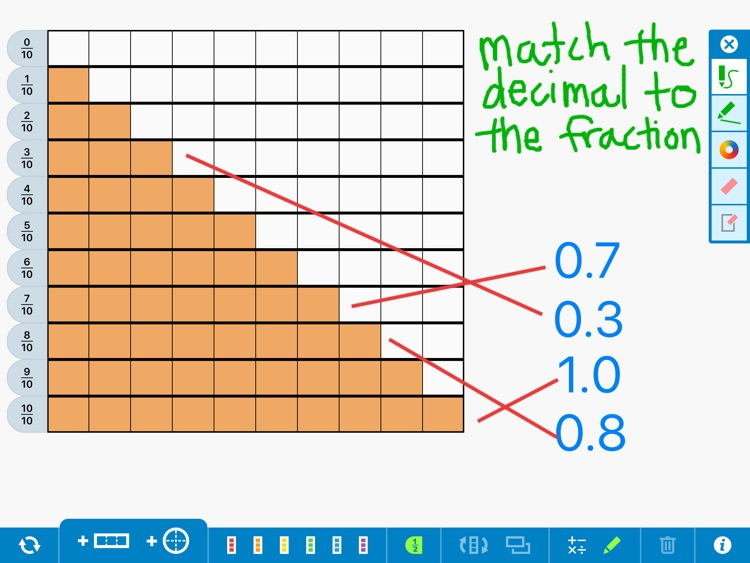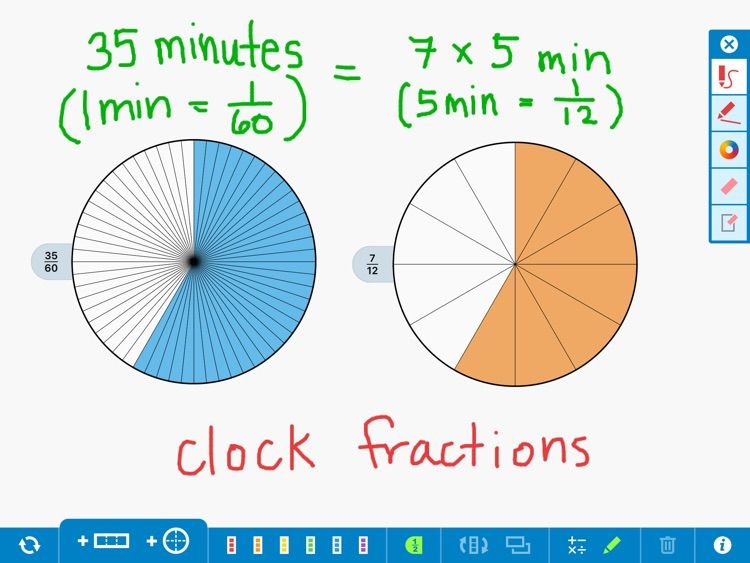### App Store Description

Fractions lets students use a bar or circle to represent, compare, and perform operations with fractions with denominators from 1 to 100. Choose the fraction model and number of equal parts. Use a color to select specific parts to show a fraction of the whole. Reveal or hide numeric labels as needed. Superimpose fractions upon each other to compare fractions or see equal parts.

FEATURES

• Use a bar or circle as the whole.

• Divide each whole into anywhere from 1 to 100 equal parts.

• Compare fractions and represent equivalent fractions.

• Add, subtract, multiply, and divide with fractions.

• Explore the relationship between fractions, percents, and decimals.

• Select the size of the whole and the number of equal parts.

• Hide and reveal fraction labels.

• Hide and reveal work with resizable covers to create your own problems and model strategies.

• Use the drawing tools to annotate work and show understanding.

• Add equations, expressions, and descriptions with the math text and writing tools.

• Share your work by saving an image or providing a share code to others.